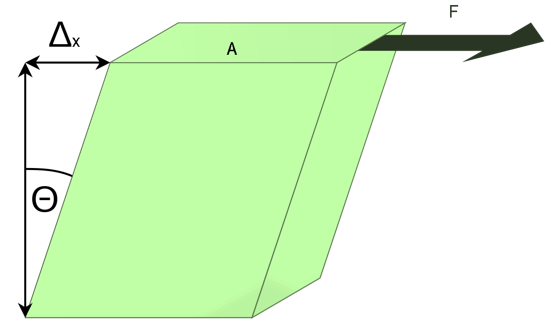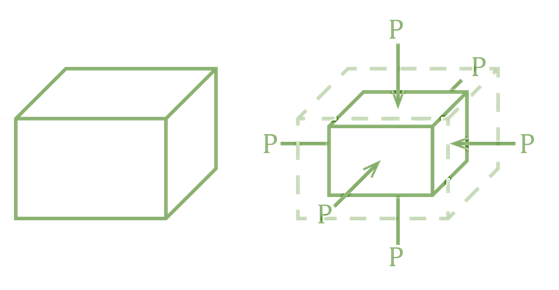GeeksforGeeks App
Open AppBrowser
Continue

## Related Articles

• CBSE Class 11 Physics Notes

A rigid body model is an idealised representation of an item that does not deform when subjected to external forces. It is extremely beneficial for evaluating mechanical systems—and many physical items are quite stiff. The degree to which an item may be regarded as stiff is determined by the physical characteristics of the material used to create it. When squashing pressures are applied to a ping-pong ball made of plastic, it becomes brittle, but a tennis ball made of rubber becomes elastic. However, in other situations, a ping-pong ball and a tennis ball may both bounce well as rigid bodies.

Similarly, someone designing prosthetic limbs may be able to mimic human limb dynamics by modelling them as rigid bodies; nevertheless, the real mix of bones and tissues is an elastic medium.

A Deformation is a change in shape caused by the application of a force. It is known that even relatively modest stresses can produce some deformation. Deformation occurs when objects or physical mediums are subjected to external pressures such as squashing, squeezing, tearing, twisting, shearing or pulling apart. The forces on objects undergoing deformation are described by two words in physics: Stress and Strain.

Stress is a measure of the magnitude of the forces that produce deformation. In general, stress is defined as force per unit area.

• Tensile stress occurs when forces draw on an object and cause it to elongate, for as when an elastic band is stretched.
• Compressive stress occurs when forces cause an item to compress.
• Bulk stress occurs when an item is pressed from all sides, such as a submarine in the depths of the ocean (or volume stress).

In some cases, the operating forces may not be tensile or compressive, yet they nevertheless cause significant deformation.

The SI Unit of Stress: Pascal (Pa). When one newton of force is applied to a one-meter-squared unit surface area, the resultant stress is one pascal.

Strain: When an object or medium is stressed, it deforms. Strain is the quantity that describes this deformation. Strain is expressed as a fractional change in length (when under tensile stress), volume (when under bulk stress), or shape (under shear stress). As a result, strain is a dimensionless quantity.

• Tensile strain is strain produced by tensile stress.
• Bulk strain (or volume strain) is strain generated by shear stress, and
• Shear strain is strain induced by shear stress.

The more the stress, the greater the strain; however, the strain-stress relationship does not have to be linear. Only when the stress is low enough is the deformation caused in direct proportion to the stress value. The elastic modulus is the proportionality constant in this relationship. The general relationship between stress and strain is shown in the linear limit of low-stress levels.

Stress = (Elastic modulus) × Strain

### Shear Modulus (η)

Shear Modulus of Elasticity is one of the mechanical characteristics of solids that may be measured. Young’s modulus and bulk modulus are two more elastic moduli. The ratio of shear stress to shear strain in a body is given by the shear modulus of material.

As shown in the figure, consider a rectangular block whose lower face is fixed and a tangential force F is applied over its upper face of area A. An equal and opposite force F comes into play on its lower fixed face. The two equal and opposite forces form a couple that exerts a torque. As the lower face of the block is fixed, the couple shears the block into parallelepiped by displacing its upper face through distance AA, I =Δx.Let AB = DC = 1 and ∠ABA’ = θ

Tangential stress = F/A

Shear Strain = θ = tan θ =AA’/AB

= Δx/l

The modulus of rigidity is given by,

η = Tangential stress/shear strain

= (F/A)/θ

=F/Aθ

=F/A .l/Δx

Units and dimensions of η: The SI unit of modulus of rigidity is Nm-2 and its CGS unit is dyne/cm2. Its dimensional formula is [ML-1T-2].

### Bulk modulus (B)

Within the elastic limit, the ratio of normal stress to the volumetric strain is called the bulk modulus of elasticity.Consider a body of volume V and surface area A .suppose a force F acts uniformly over the whole surface of the body and it decreases the volume by  ΔV as shown in the figure. Then bulk modulus of elasticity is given by

B = normal stress/volumetric strain

= -F/A/ΔV/V

B=-F/A.V/ΔV = -p.V/ΔV

where p (=F/A) is the normal pressure. A negative sign shows that the volume decreases with the increase in stress.

Units and dimensions of B: The SI unit of the bulk modulus is N/m2 or Pascal (Pa) and its CGS unit are done/cm2. Its dimensional formula is [ML-1 T-2]

Compressibility: The reciprocal of the bulk modulus of a material is called its compressibility.

Compressibility =1/B

The SI unit of compressibility is N-1m2 and the cgs unit of compressibility is dyne-1cm2

### Sample Problems

Question 1: A uniform wire of Steel of length 2.5 m and density 8.0 g/cm-3 weighs 50 g. When stretched by a force of 10 kg, the length increases by 2 mm. Calculate Young’s modulus of steel.

Given l=2.5 m =250 cm

Δl =2 mm =0.2 cm

F=10 kgf =10 ×9.8 N =10 ×9.8 ×105 dyne

Mass =volume  × density

A =Mass/l ×ρ =50/250 ×8 =0.025 cm2

Young’s modulus  =F/A . l/Δl

=10 ×9.8 ×105 ×250/0.025 ×0.2

=4.9 ×1011 dyne cm -2

Question 2: What is the percentage increase in the length of a wire of diameter 2.5 mm stretched by a force of 100 kg wt? Young’s modulus of elasticity of the wire is 12.5  ×10^11 dyne cm -2

Given r  = 1.25 mm= 0.125 cm

F =100 ×9.8  =980 N=98×10^6 dyne

Y= 12.5 ×10^11dyne cm ^- 2

Y=F/A.l/Δl or Δl/l =F/AY =F/πr2Y

The percentage increase in length is

Δl/l ×100 =F×100/πr2 Y

=98× 106× 7 ×100 /22 ×(0.125)2 ×12.5×1011

=15.965×10-2

=0.16%

Question 3: Calculate the increase in pressure required to decrease the volume of a water sample by 0.01% . Given that the bulk modulus of water is 2.2 ×10 9 Pa.

Here, fractional decrease  in volume

ΔV/V = -0.01% =  -0.01/100 =-10-4

Bulk modulus of water B=2.2 ×10 9 Pa

Bulk modulus  B =P/ΔV/V

P = -B.ΔV/V = -(2.2 ×109). (-10 -4)

=2.2 ×105 Pa

Question 4: Find the change in volume which 1m3 of water will undergo when taken from the surface to the bottom of a lake 100 m deep. Given volume elasticity of water is 22,000 atmosphere.

Here V =1m3, h=100 m, g=9.8 ms -2

ρ(water) =1000 kg m -3

P = hρh =100 ×1000 ×9.8 =9.8 ×10^5Nm^-2

B=22000 atm =22000 ×1.013×10^5 Nm^-2

=22.286×10^8Nm^-2

ΔV =pV/B =9.8×105 ×1/22.286 ×108

=4.4 ×10^-4 m3

Question 5: An Indian rubber cube of side 7 cm has one side fixed while tangential force equal to the weight of 200 kg is applied to the opposite face. Find the shearing strain produced and the distance through which the strained side moves. modulus of rigidity for rubber is 2 ×10 dyne cm-2

Here ,l=7 cm ,F =200 kg f=200 ×1000 ×981 dyne

η =2 ×20^7 dyne cm-2

Area of the free face,

A =l2 =7 cm × 7 cm =49 cm2

η =F/Aθ

θ =F/Aη =200 × 1000 × 981 /49 × 2 ×10^7